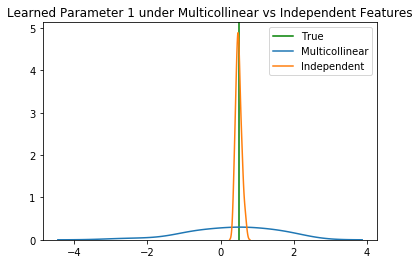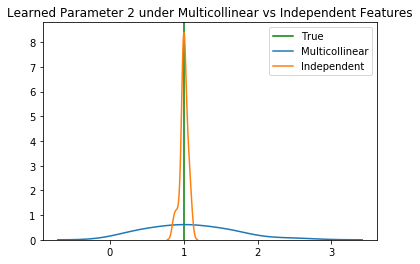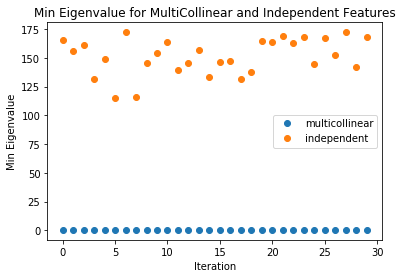# The Problem of Multicollinearity in Linear Regression

Here we’ll talk about multicollinearity in linear regression.
This occurs when there is correlation among features, and causes the learned model to have very high variance. Consider for example predicting housing prices. If you have two features: number of bedrooms and size of the house in square feet, these are likely to be correlated. Consider a correctly specified model for linear regression. The mean squared error in the learned modelcan be decomposed into squared bias and variance. If we estimatevia ordinary least squares (OLS), the bias is, so that

(1)Let’s investigate the effect of multicollinearity by looking at parameter learning accuracy under both multicollinear features and independent features. We will first look at empirical performance and then talk about the theory of why we see this. We will use the following linear modelwherei.i.d. and. For multicollinear data we use

(2)Thus the two features have some amount of multi-collinearity, but the gram matrixis still invertible. For independent data we use

(3)We now sample 200pairs of both multicollinear and independent data and perform OLS.  We repeat this 30 times and plot density estimates of the learned parameters.As we can see, the variance with multicollinear features is drastically higher.  In particular, with independent features we generally get a pretty good learned model, whereas with multicollinear features, some of the learned models are useless. Why is this? Recall that for a given dataset,

(4)Now with multicollinearity,will have “almost” linearly dependent columns, leading to some eigenvalues being very small. Now

(5)whereis theth eigen-value. Thus having a single small eigenvalue will lead to very large mean squared parameter error. Let’s see what the smallest eigenvalues look like in practice under both the multicollinear and independent data.As expected, the min eigenvalues are much smaller under multicollinearity. To sum up:

1. Multicollinearity is when features are correlated
2. It leads to high variance in the learned model.
3. The reason for this is that the the min eigenvalues of the Gram matrix of the features becomes very small when we have multicollinearity.
In the future, we will discuss how to detect multicollinearity and what to do about it.

This site uses Akismet to reduce spam. Learn how your comment data is processed.